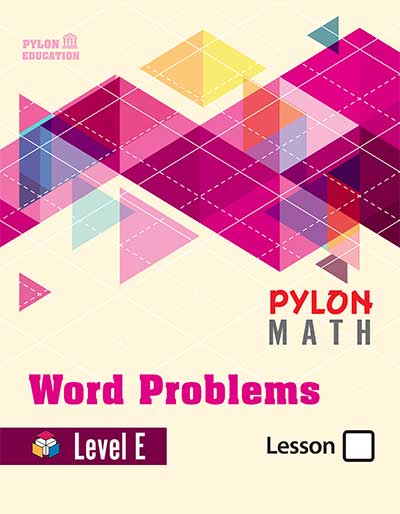# Word Problem Level E1. ### Place Value

• Place Value
• Comparing Decimals
• Subtracting Decimals
2. ### Fractions

• Parts of a Whole
• Equivalent Fractions
• Simplifying Fractions
• Mixed Numbers
• Improper Fractions
• Problem Solvings

• Unlike Denominators
• Factors
• Greatest Common Factors
• Least Common Multiples
• Least Common Denominators
• Problem Solvings
4. ### Subtracting Fractions

• Subtracting Proper Fractions
• A Whole Number and a Fraction
• Subtracting Improper Fractions
• Problem Solvings
5. ### Multiplying Fractions

• Multiplying Fractions
• Reducing Fractions
• Mixed Fractions
• Problem Solvings
1. ### Dividing Fractions

• Dividing Simple Fractions
• Dividing Mixed Numbers
• Problem Solvings
2. ### Decimals

• Decimals and Fractions
• Multiplying Decimals
• Multiplying by 10,100,1000
• Problem Solvings
3. ### Dividing Decimals

• Dividing Decimals
• Placing Decimal Points
• Problem Solvings
4. ### Geometry and Other Topics

• Areas
• Volumes
• Rounding Places
• Mean, Median, Mode
• Probability
5. ### Graphs

• Bar Graphs and Pictographs
• Line Graphs, Line Plots
• Stem-and-Leaf Plots, Circle Graph
• Data Analysis,Pictographs
• Reading Graphs, Double Line Graphs
• Properties, Ordered Pairs